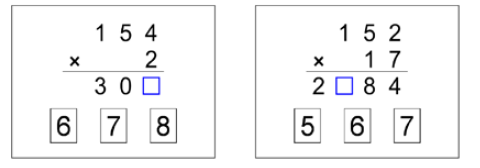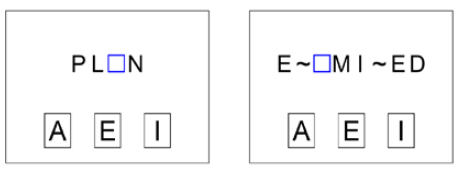# Math Anxiety and Math Avoidance

Authors: Kyoung Whan Choe, Jalisha B. Jenifer, Christopher S. Rozek, Marc. G. Berman, Sian L. Bellock

First author’s institution: The University of Chicago

Journal: Science Advances 5, eaay1062 (2019)

When I introduce myself as a physicist to a non-scientist, it isn’t uncommon for the other person to respond with something about being interested in physics but finding the math too difficult when they took a physics course or not liking the math that goes along with science (though a reference to The Big Bang Theory is usually the first thing to come up). Unfortunately, concerns about math are widespread as various studies have reported (and summarized here), with estimates of its prevalence ranging from between 30%-90% of adults being affected by some form of math anxiety. Many studies have assumed that people with math anxiety then engage in math avoidance behaviors such as taking fewer math-related courses or pursuing careers involving high amounts of math.

Yet, there has been little experimental evidence linking math anxiety to math avoidance. Previous studies trying to establish the link have used math course enrollment or math test-taking strategies as measures of math avoidance, but both of these measures are related to math ability. Therefore, if someone experiences math anxiety but also thinks they are “bad at math”, is it their anxiety or their belief about their math ability that causes the math avoidance?

Today’s study uses a different approach to work around the effects of perceived math ability. The authors used research on motivated behavior, which hypothesizes that people make decisions based on a series of cost-benefit evaluations regarding the effort of the task, to create a task where the benefit and reward could be easily changed. If people with high math anxiety do engage in math avoidance, then even if the expected reward for doing a more challenging math task is much higher than doing an easier math task, people with math anxiety should still prefer to do the easier math task.

The actual task consisted of participants recruited from Turk Prime answering math problems of varying difficultly for a small cash prize. The participant first chose whether they wanted to answer an “easy” math problem for 2 cents or a “hard” math problem for anywhere between 2 and 6 cents. The participant was told how much the “hard” problem was worth before making their selection. After selecting the problem, the participant had 7 seconds to answer the question. Each math problem required the participant to select 1 of 3 possible answers to fill in the missing digit in the answer to a math problem (see figure 1).Figure 1: Sample easy (left) and hard (right) math problems. Participants had to select one of the three options to fill in the blue box. (Adapted from figure 1).

Each participant then completed two blocks of 50 trials. After each block, the participants performed a similar set of trials but instead of math problems, the participants selected which of 3 letters would complete an English word with a letter missing (figure 2).Figure 2: Sample easy (left) and hard (right) word problems. Participants had to select one of the three options to fill in the blue box. “~” represents letters that were excluded to increase the challenge of the problem. (Adapted from figure 1)

To ensure that math ability did not affect their results, the researchers conducted a validation study to determine the difficulty of their math and word problems. Since the task was computerized, the researchers made the test adaptive so that all participants would score around 70% correct on the hard problems as had been determined by the validation study. That is, if someone consistently answered hard math or word problems incorrectly, the next hard problem would be one that most participants in the validation study had answered correctly.

Since each participant was expected to score around 70% correct on the hard problems (and nearly 100% on the easy problems), the researchers could quantify whether the easy or hard problem had a higher expected reward. If the reward for the hard problem was at least 4 cents, a rational participant should always pick the hard problem since they will earn more money. However, if the participant is not “rational” and instead making a decision influenced by their math anxiety, they would not choose a hard math question, even if it would earn them more money.

To quantify the level of math avoidance of the participants, the participants completed the Mathematics Anxiety Rating Scale where they responded to how anxious they would feel in various situations. To quantify the level of math avoidance, the researchers calculated the probability the participant selected a hard problem, which was the number of hard problems selected divided by the total number of problems attempted.

As the title of the paper suggests, the level of math anxiety was negatively correlated with the probability of the participant selecting a hard math problem (figure 3). However, math anxiety was not correlated with the probability of selecting a hard word problem, suggesting that participants were trying to maximize their earnings and not just answering easy questions. The researchers then confirmed this result using a linear mixed-effect model, which found there was a significant interaction between math anxiety and the domain of the problem (math or word).Figure 3: Plots of math anxiety vs the probability of choosing a hard problem for both math (left) and word (right) problems. Math anxiety was negatively correlated with choosing hard math problems, but not hard word problems. (Adapted from figure 3.)

Perhaps this study had some of the same issues as previous studies though. For example, maybe people with higher math anxiety thought they weren’t “good at math” and since the participants only earned the reward if they answered the question correctly, they may have again avoided harder math questions due to their perceived ability. Yet, math anxiety was also associated with lower performance on word problems, but participants did not avoid hard word problems, suggesting that ability to solve difficult problems could not be an alternative explanation.

Alternatively, maybe 7 seconds wasn’t enough time to solve the difficult problems, and hence those with higher math anxiety avoided these problems because they didn’t think they could solve the problems in time. Surprisingly, participants with higher levels of math anxiety spent less time on hard math problems than participants with lower levels of math anxiety, suggesting that time was not a factor.

Finally, the researchers suggested that maybe math anxiety was a specific instance of some larger form of anxiety such as test anxiety or trait anxiety. However, when using various scales to assess these other forms of anxiety and controlling for these in a linear mixed-effects model, math anxiety was still negatively associated with the probability of choosing a hard math problem.

Since none of the alternative explanations could account for the link between math anxiety and avoiding the hard math problems, the researchers reasoned that math anxiety must be causing the participants to avoid the harder problems.

Overall, the study represents the first experimental evidence linking math anxiety and math avoidance. While this paper claims that addressing the math anxiety-avoidance link may result in increased interest and success in STEM careers, this study did not actually use students or math representative of what would be found in even an introductory STEM course. While reducing math anxiety is still worthwhile, we should be cautious about using the results of this study to suggest that reducing math anxiety may lead to increased enrollment in STEM majors/careers.

Figures used under CC BY 4.0. Header image from Flickr User Jimmie used under CC BY 2.0.